5.2 Constructive and Destructive Interference

In the last section we discussed the fact that waves can move through each other, which means that they can be in the same place at the same time. This is very different from solid objects. Thus, we need to know how to handle this situation. As it turns out, when waves are at the same place at the same time, the amplitudes of the waves simply add together and this is really all we need to know! However, the consequences of this are profound and sometimes startling.

To start exploring the implications of the statement above, let’s consider two waves with the same frequency traveling in the same direction: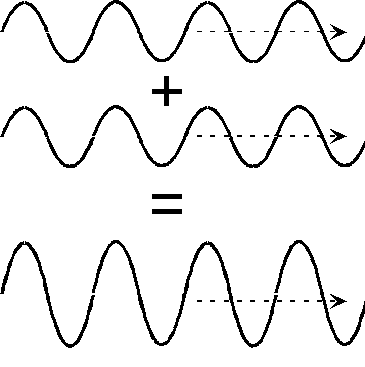If we add these two waves together, point-by-point, we end up with a new wave that looks pretty much like the original waves but its amplitude is larger. This situation, where the resultant wave is bigger than either of the two original, is called constructive interference. The waves are adding together to form a bigger wave. You may be thinking that this is pretty obvious and natural – of course the sum of two waves will be bigger than each wave on its own. However, carefully consider the next situation, again where two waves with the same frequency are traveling in the same direction: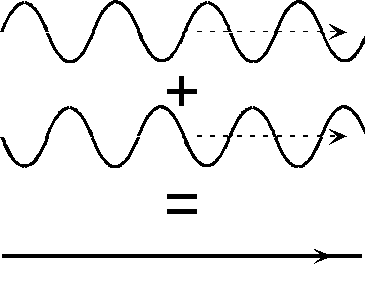Now what happens if we add these waves together? When the first wave is up, the second wave is down and the two add to zero. When the first wave is down and the second is up, they again add to zero. In fact, at all points the two waves exactly cancel each other out and there is no wave left! This is the single most amazing aspect of waves. The sum of two waves can be less than either wave, alone, and can even be zero. This is called destructive interference.

When the peaks of the waves line up, there is constructive interference. Often, this is describe by saying the waves are "in-phase". Although this phrase is not so important for this course, it is so commonly used that I might use it without thinking and you may hear it used in other settings. Similarly, when the peaks of one wave line up with the valleys of the other, the waves are said to be "out-of-phase". Phase, itself, is an important aspect of waves, but we will not use this concept in this course.

How could we observe this difference between constructive and destructive interference. Given the fact that in one case we get a bigger (or louder) wave, and in the other case we get nothing, there should be a pretty big difference between the two. We will explore how to hear this difference in detail in Lab 7.

The most important requirement for interference is to have at least two waves. One wave alone behaves just as we have been discussing. We shall see that there are many ways to create a pair of waves to demonstrate interference. The simplest way to create two sound waves is to use two speakers. If we place them side-by-side, point them in the same direction and play the same frequency, we have just the situation described above to produce constructive interference: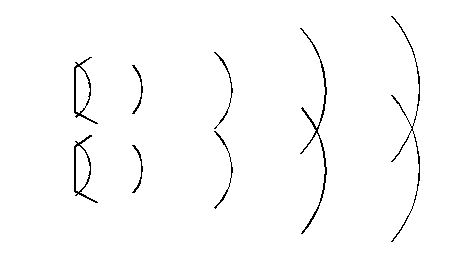If we stand in front of the two speakers, we will hear a tone louder than the individual speakers would produce. The two waves are in phase. Now imagine that we start moving on of the speakers back: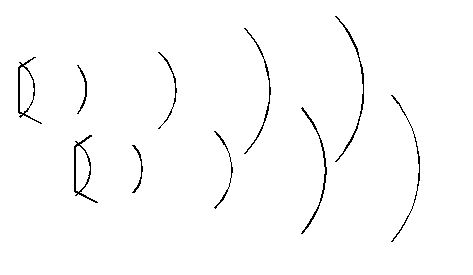At some point, the two waves will be out of phase – that is, the peaks of one line up with the valleys of the other creating the conditions for destructive interference. If we stand in front of the speakers right now, we will not hear anything! This must be experienced to really appreciate. Equally as strange, if you now block one speaker, the destructive interference goes away and you hear the unblocked speaker. In other words, the sound gets louder as you block one speaker!

How far back must we move the speaker to go from constructive to destructive interference? We know that the distance between peaks in a wave is equal to the wavelength. If we look back at the first two figures in this section, we see that the waves are shifted by half of a wavelength. So, in the example with the speakers, we must move the speaker back by one half of a wavelength.

What happens if we keep moving the speaker back? At some point the peaks of the two waves will again line up: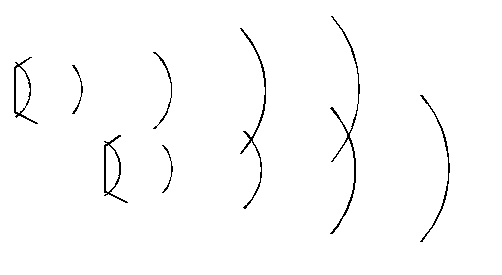At this position, we will again have constructive interference! As the speaker is moved back the waves alternate between constructive and destructive interference.

What the example of the speakers shows is that it is the separation of the two speakers that determines whether there will be constructive or destructive interference. If the speakers are at the same position, there will be constructive interference at all points directly in front of the speaker. If the speakers are separated by half a wavelength, then there is destructive interference, regardless of how far or close you are to the speakers. However, it already has become apparent that this is not the whole story, because if you keep moving the speaker you again can achieve constructive interference. This can be fairly easily incorporated into our picture by saying that if the separation of the speakers in a multiple of a wavelength then there will be constructive interference. Note that zero separation can always be considered a multiple of a wavelength.

What about destructive interference? We know that if the speakers are separated by half a wavelength there is destructive interference. However, if we move an additional full wavelength, we will still have destructive interference. So, this case is a bit hard to state, but if the separation is equal to half a wavelength plus a multiple of a wavelength, there will be destructive interference. Thus, we have described the conditions under which we will have constructive and destructive interference for two waves with the same frequency traveling in the same direction. Unfortunately, the conditions have been expressed in a cumbersome way that is not easily applied to more complex situations. So, before going on to other examples, we need a more mathematically concise way of stating the conditions for constructive and destructive interference.

The proper way to define the conditions for having constructive or destructive interference requires knowing the distance from the observation point to the source of each of the two waves. Since there must be two waves for interference to occur, there are also two distances involved, R1 and R2. For two waves traveling in the same direction, these two distances are as follows: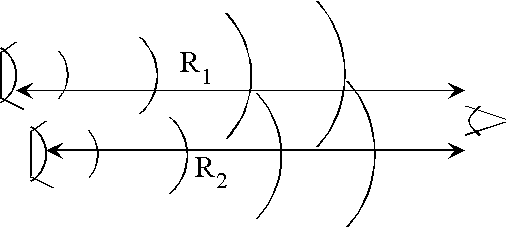When we discussed interference above, it became apparent that it was the separation between the two speakers that determined whether the interference was constructive or destructive. From this diagram, we see that the separation is given by R1 – R2. So, really, it is the difference in path length from each source to the observer that determines whether the interference is constructive or destructive. I emphasize this point, because it is true in all situations involving interference. The only difficulty lies in properly applying this concept.

With this more rigorous statement about interference, we can now right down mathematically the conditions for interference:

Constructive interference:

We saw that when the two speakers are right next to each other, we have constructive interference. However, if the speakers are next to each other, the distance from each to the observer must be the same, which means that R1 = R2. Or, we can write that R1 - R2 = 0. I.e. the path difference must be equal to zero. But, we also saw that if we move one speaker by a whole wavelength, we still have constructive interference. Remember that we use the Greek letter l for wavelength. With this, our condition for constructive interference can be written:

R1 – R2 = 0 + nl .

Here, the variable n is used to specify an integer and can take on any value, as long as it is an integer. This ensures that we only add whole numbers of wavelengths.

Destructive interference:

Once we have the condition for constructive interference, destructive interference is a straightforward extension. The basic requirement for destructive interference is that the two waves are shifted by half a wavelength. This means that the path difference for the two waves must be: R1 – R2 = l /2. But, since we can always shift a wave by one full wavelength, the full condition for destructive interference becomes:

R1 – R2 = l /2 + nl .

Now that we have mathematical statements for the requirements for constructive and destructive interference, we can apply them to a new situation and see what happens.

Waves with the same frequency traveling in opposite directions.To create two waves traveling in opposite directions, we can take our two speakers and point them at each other, as shown in the figure above. We again want to find the conditions for constructive and destructive interference. As we have seen, the simplest way to get constructive interference is for the distance from the observer to each source to be equal. Using our mathematical terminology, we want R1 – R2 = 0, or R1 = R2. Looking at the figure above, we see that the point where the two paths are equal is exactly midway between the two speakers (the point M in the figure). At this point, there will be constructive interference, and the sound will be strong.

It makes sense to use the midpoint as a reference, as we know that we have constructive interference. How far must we move our observer to get to destructive interference? If we move to the left by an amount x, the distance R1 increases by x and the distance R2 decreases by x. If R1 increases and R2 decreases, the difference between the two R1 – R2 increases by an amount 2x. So, at the point x, the path difference is R1 – R2 = 2x. Now comes the tricky part. If 2x happens to be equal to l /2, we have met the conditions for destructive interference. Therefore, if 2x = l /2, or x = l /4, we have destructive interference. To put it another way, in the situation above, if you move one quarter of a wavelength away from the midpoint, you will find destructive interference and the sound will sound very weak, or you might not hear anything at all.

What happens if we keep moving our observation point? If the path difference, 2x, equal one whole wavelength, we will have constructive interference, 2x = l . Solving for x, we have x = l /2. In other words, if we move by half a wavelength, we will again have constructive interference and the sound will be loud.

As we keep moving the observation point, we will find that we keep going through points of constructive and destructive interference. This is a bit more complicated than the first example, where we had either constructive or destructive interference regardless of where we listened. In this case, whether there is constructive or destructive interference depends on where we are listening. However, the fundamental conditions on the path difference are still the same.

What does this pattern of constructive and destructive interference look like? We can map it out by indicating where we have constructive (x) and destructive (· ) interference: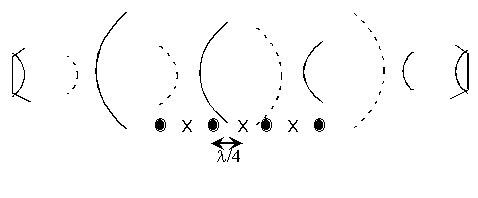What we see is a repeating pattern of constructive and destructive interference, and it takes a distance of l /4 to get from one to the other. Where have we seen this pattern before? At a point of constructive interference, the amplitude of the wave is large and this is just like an antinode. At a point of destructive interference, the amplitude is zero and this is like an node. So, if we think of the point above as antinodes and nodes, we see that we have exactly the same pattern of nodes and antinodes as in a standing wave. From this, we must conclude that two waves traveling in opposite directions create a standing wave with the same frequency! You can get a more intuitive understanding of this by looking at the Physlet entitled Superposition.

Translating the interference conditions into mathematical statements is an essential part of physics and can be quite difficult at first. Moreover, a rather subtle distinction was made that you might not have noticed. On the one hand, we have some physical situation or geometry. This refers to the placement of the speakers and the position of the observer. This really has nothing to do with waves and it simply depends on how the problem was set up. Given a particular setup, you can always figure out the path length from the observer to the two sources of the waves that are going to interference and hence you can also find the path difference R1 – R2.

On the other hand, completely independent of the geometry, there is a property of waves called superposition that can lead to constructive or destructive interference. We can express these conditions mathematically as:

R1 – R2 = 0 + nl , for constructive interference, and
R1 – R2 = l /2 + nl for destructive interference.

Again, R1 – R2 was determined from the geometry of the problem. These two aspects must be understood separately: how to calculate the path difference and the conditions determining the type of interference.

Only then should these to aspects be combined to determine whether there is constructive or destructive interference at a particular location of the observer. This can be summarized in a diagram, using waves traveling in opposite directions as an example: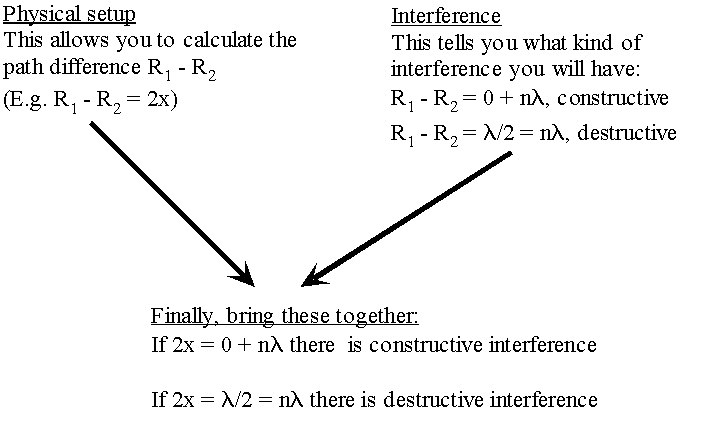In the next sections, we will explore many more situations for seeing constructive and destructive interference.